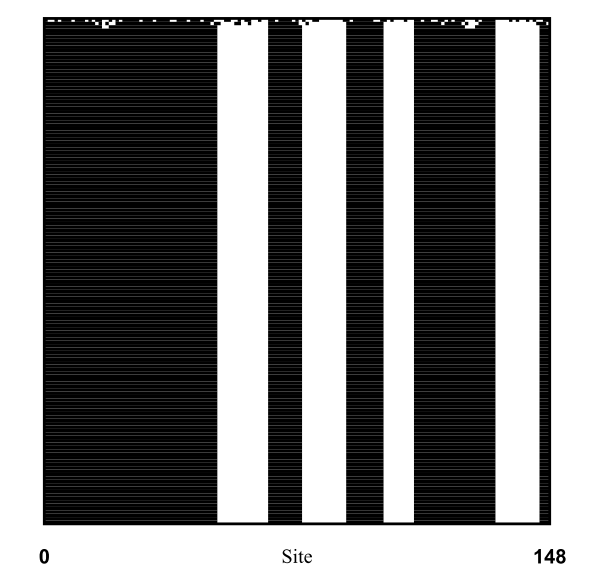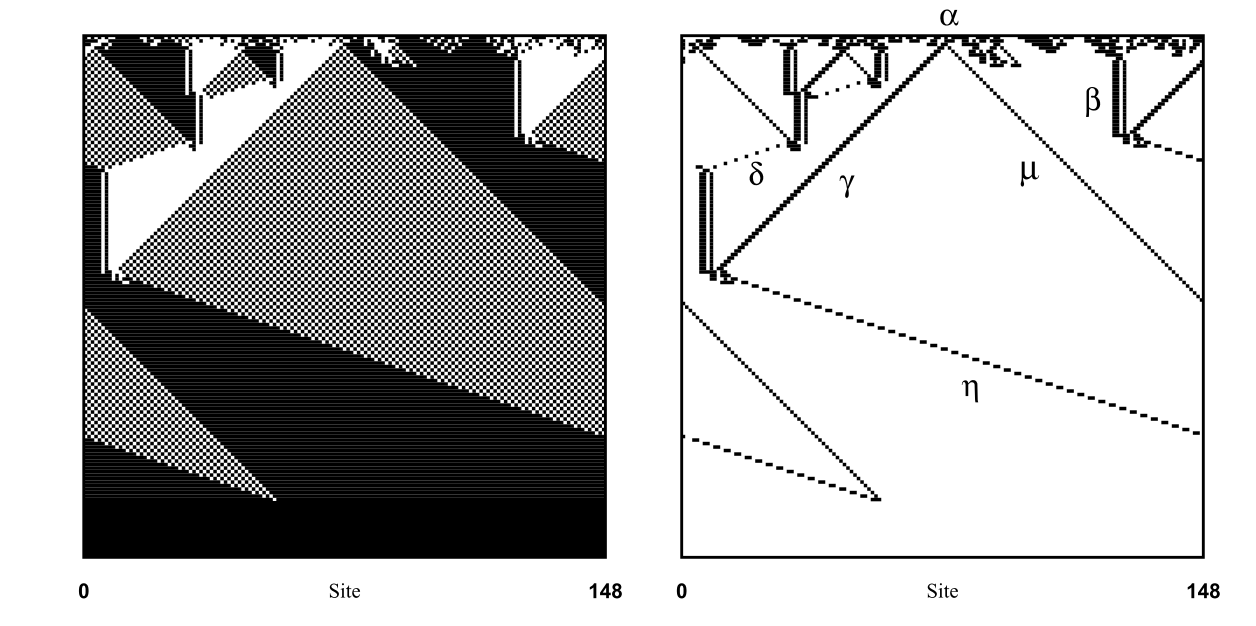## Evolving Cellular Automata with Genetic Algorithms

Mitchell, M., Crutchfield, J., & Das, R. (1996). Evolving cellular automata with genetic algorithms: A review of recent work. Proceedings of the International Conference on Evolutionary Computing and Its Applications, 1, 1–14.

This paper investigates how evolution can create emergent computation, in which simple components that are only locally connected and informed can result in global information processing capabilities.

1D binary cellular automata (CAs) are used:

• the universe consists of N cells in a row; cells are either dead or alive (0 or 1)
• Initial Conditions (ICs) are updated according to the CA ruleset $~\phi$.
• $\phi$ can be expressed as a table determining the output bit given a cell and its radius of r neighbours.
• the universe is circular (1D toroidal), meaning that the end loops and connects to the start.

A CA is said to be performing a computation if some input can be encoded into an IC, and the CA transforms this IC into some output configuration which can be decoded into a meaningful result.

### Density Classification

• The goal in the density classification task is for the CA to decide if the starting configuration contains a majority of living or dead cells.
• Denoting $\rho$ as the density of 1s, this task is more precisely called the $\rho_c = 1/2$ task, where the cutoff is a simple majority of 50%.
• If $\rho > \rho_c$, then within M iterations the configuration of the CA should be all 1s, and if $\rho < \rho_c$ then it should be all 0s.
• The difficulty of this problem is that each cell only has local knowledge, but the 1s in the IC can be spread across the universe, so some transfer of information must occur.
• Using a radius $r=3$, each cell has a 7-bit input (itself and the three neighbours on either side).
• A naive first approximation would be to use the majority rule, in which the output bit is the simple majority of the 7-bit input.
• We can visualise the CA’s life with a space-time diagram, which plots the configuration at each iteration (going down the page). The space-time diagram for the majority rule shows that it does not perform the $\rho_c = 1/2$ task:## Evolution of Cellular Automata

• If $r=3$, there are $2^7 = 128$ rules and $2^{128}$ possible mappings (each rule can have an output of 0 or 1), far too many for a brute force search.
• This paper uses $N=149$, though any reasonably large odd number should work.
• Each chromosome is a ruleset – the initial population consists of 100 chromosomes sampled from a distribution that is uniform over the density of 1s in the output bits.
• Fitness is computed by iterating each chromosome on 100 random initial ICs (sampled such that exactly half have $\rho < \rho_c$) for up to $M=2N$ steps. The fitness F of a rule is defined as the fraction of ICs for which that rule produced the correct behaviour (all 1s or 0s after M steps – no partial credit).
• The ICs must be generated by sampling from a uniform distribution, because an unbiased sample (binomial) has a strong peak at $\rho = 0.5$, which is the hardest to classify.
• Evolution:
• each generation uses 100 random ICs
• the 100 CA rules are ranked in order of fitness
• the top 20 rules (the elites) are copied into the next generation
• the other 80 rules in the next generation are formed by single-point crossovers between randomly chosen elites (with replacement) which are then mutated in two random places.

## Results

• 300 runs were performed. Performance is measured over 10,000 random ICs from an unbiased sample (i.e many will have $\rho \approx 0.5$).
• Many evolved a strategy that expands blocks of adjacent 1s and 0s. This $\phi_{exp}$ strategy does not scale well for large N, and is not a good example of emergent computation.
• One of the problems was overfitting to $N=149$ or to easy ICs. This is partially because of the uniform sampling, thus there needs to be a compromised between easy and hard training examples.
• However, some of the runs resulted in a sophisticated strategy which looks can be explained in terms of regular domains (regions that are computationally homogeneous), and particles (the boundaries of these domains).## Extensions

• This approach can also be used for the spontaneous synchronisation task, in which any IC should result in alternating all 1 / all 0 configurations.
• It is possible that CAs could be evolved to perform complex image processing tasks, using genetic algorithms.
• These models may give insight into how global decentralised computation could emerge from simple components.